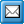﻿ Graphical Properties in QPR Modeling Client's XML Export
QPR Knowledge Base 2017.1

Graphical Properties in QPR Modeling Client's XML ExportGraphical Properties in QPR Modeling Client's XML ExportThe XML Export in QPR Modeling Client supports the export of basic graphical properties such as colors, size and position of an element, flow's source and target level as well as the modeling direction of the diagram.

In order to have the graphical properties exported, the integration settings files (see chapter Integration Settings Files) need to have the [parameters] section key ExportGraphicalProperties=true. This setting is not asked in the Export Wizard, so it must be input manually into the settings files.

An example of graphical properties in XML (the width, height and position of an instance):

<Attribute AttributeName="Instance graphical properties">

<Record>

<Field Name="InstanceId" Value="1"/>

<Field Name="ElementWidth" Value="80"/>

<Field Name="ElementHeight" Value="40"/>

<Field Name="ElementPosX" Value="-100"/>

<Field Name="ElementPosY" Value="0"/>

</Record>

</Attribute>

An example of additional instance properties for flows (source and target levels and elements together with their positions):

<Value>

LevelId=88;From.ElementId=418;From.InstanceId=1;To.ElementId=0;To.InstanceId=0;From.PosX=280;From.PosY=265;To.PosX=310;To.PosY=-130;

</Value>

<Value>

LevelId=45;From.ElementId=88;From.InstanceId=1;To.ElementId=444;To.InstanceId=1;From.PosX=30;From.PosY=315;To.PosX=112;To.PosY=225;

</Value>

</Attribute>

An example of additional instance properties for organization items (diagram ID and the start and end positions):

<Value>

LevelId=1456498602;Start.Pos=194;End.Pos=424;

</Value>

<Value>

LevelId=47;Start.Pos=1;End.Pos=121;

</Value>

<Value>

LevelId=835121058;Start.Pos=596;End.Pos=696;

</Value>

<Value>

LevelId=665265220;Start.Pos=380;End.Pos=488;

</Value>

<Value>

LevelId=930664942;Start.Pos=160;End.Pos=260;

</Value>

</Attribute>